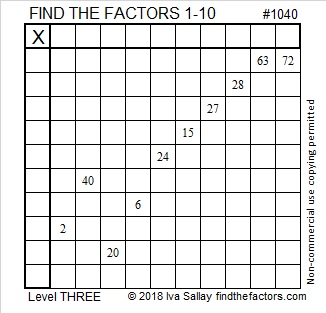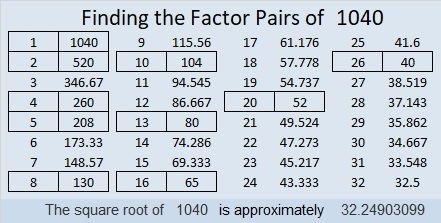# 1040 and Level 3

See clues 63 and 72 near the top of this puzzle? Start there and work down cell by cell to find all the factors that will make this puzzle become a multiplication table. Only write each number from 1 to 10 once in the top row and once in the first column.Print the puzzles or type the solution in this excel file: 10-factors-1035-1043

Now here are some facts about the number 1040:

• 1040 is a composite number.
• Prime factorization: 1040 = 2 × 2 × 2 × 2 × 5 × 13, which can be written 1040 = 2⁴ × 5 × 13
• The exponents in the prime factorization are 4, 1 and 1. Adding one to each and multiplying we get (4 + 1)(1 + 1)(1 + 1) = 5 × 2 × 2 = 20. Therefore 1040 has exactly 20 factors.
• Factors of 1040: 1, 2, 4, 5, 8, 10, 13, 16, 20, 26, 40, 52, 65, 80, 104, 130, 208, 260, 520, 1040
• Factor pairs: 1040 = 1 × 1040, 2 × 520, 4 × 260, 5 × 208, 8 × 130, 10 × 104, 13 × 80, 16 × 65, 20 × 52 or 26 × 40
• Taking the factor pair with the largest square number factor, we get √1040 = (√13)(√65) = 4√65 ≈ 32.24903.1040 is the sum of the twelve prime numbers from 61 to 109.
It is also the sum of these four prime numbers:
251 + 257 + 263 + 269 = 1040

1040 is the hypotenuse of FOUR different Pythagorean triples:
256-1008-1040 which is 16 times (16-63-65)
400-960-1040 which is (5-12-13) times 80
528-896-1040 which is 16 times (33-56-65)
624-832-1040 which is (3-4-5) times 208

I like the way 1040 looks in a couple of other bases:
It’s 2020 in BASE 8 because 2(8³) + 2(8) = 2(520) = 1040, and
it’s palindrome 3A3 in BASE 17 (A is 10 base 10) because 3(17²) + 10(17) + 3(1) = 1040

This site uses Akismet to reduce spam. Learn how your comment data is processed.